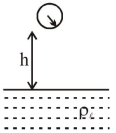# In an experiment to verify Stokes law, a small spherical ball

Question:

In an experiment to verify Stokes law, a small spherical ball of radius $\mathrm{r}$ and density $\rho$ falls under gravity through a distance $\mathrm{h}$ in air before entering a tank of water. If the terminal velocity of the ball inside water is same as its velocity just before entering the water surface, then the value of $\mathrm{h}$ is proportional to :

(ignore viscosity of air)

1. $\mathrm{r}$

2. $r^{4}$

3. $\mathrm{r}^{3}$

4. $\mathrm{r}^{2}$

Correct Option: , 2

Solution:After falling through $\mathrm{h}$, the velocity be equal to terminal velocity

$\sqrt{2 \mathrm{gh}}=\frac{2}{9} \frac{\mathrm{r}^{2} \mathrm{~g}}{\eta}\left(\rho_{\ell}-\rho\right)$

$\Rightarrow \mathrm{h}=\frac{2}{81} \frac{\mathrm{r}^{4} \mathrm{~g}\left(\rho_{\ell}-\rho\right)^{2}}{\eta^{2}}$

$\Rightarrow \mathrm{h} \propto \mathrm{r}^{4}$